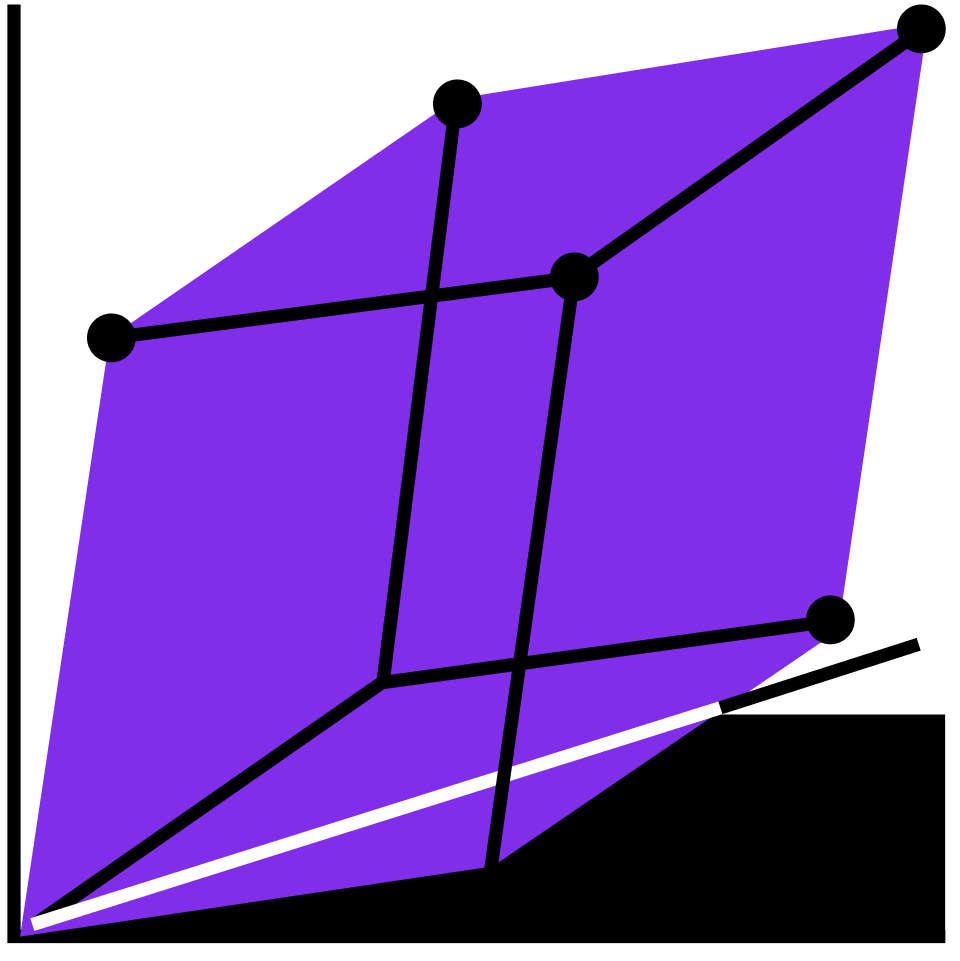## 4.1 Introduction to Linear Algebra

Get familiar with matrices, vectors, and more as you explore the theory and real-world applications of linear algebra.

Two Linear Equations in Two Unknowns

Three Unknowns

Gaussian Elimination

The Full Story of Gaussian Elimination

Application: Kirchhoff and Circuits

Vector Spaces

Subspaces and Span

Linear Independence

Basis and Dimension

Dot Products and Inner Products

Least Squares

Matrix Algebra

Inverses and Systems of Equations

Four Fundamental Subspaces

Linear Transformations

Properties of Linear Transformations

2x2 Determinants

Determinants in Higher Dimensions

Representation by a Matrix

Change of Basis

Polynomial Interpolation

Eigenvalues and Eigenvectors

Characteristic Polynomial

Diagonalizability

PageRank and Exponentiation

### Course description

Linear algebra is pervasive in just about all modern scientific subjects, including physics, mathematics, computer science, electrical engineering, economics, and aeronautical engineering. You’ll learn about its applications in computer graphics, signal processing, machine learning, RLC circuit analysis, and control theory. By the end of this course, you’ll be able to solve systems of equations of all flavors and complexities using linear algebra, from a simple 2x2 matrix equation to much more complex systems involving many variables.

### Topics covered

• Bases
• Determinants
• Diagonalizable Matrices
• Dot products
• Eigenvalues and Eigenvectors
• Gaussian Elimination
• Inverses
• Linear Independence
• Linear Transformations
• Matrices
• Subspaces
• Vector Spaces

### Prerequisites and next steps

A basic understanding of calculus and linear equations is necessary.